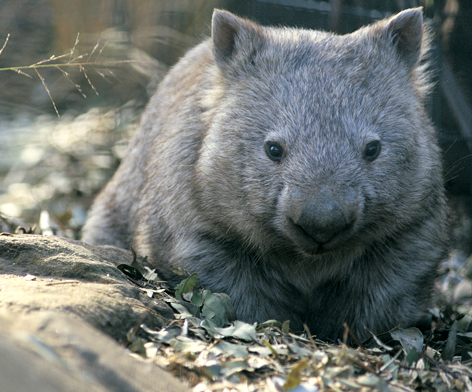# Math 191.

The goal of this course is expose students to techniques in combinatorial topology. Historically, the first topological invariants used by mathematicians were from combinatorial construction. More recently, combinatorial topology has been used to attack problems in knot topology, partially ordered sets, polytope and matroid theory. Open problems in combinatorial topology are easily stated, and provide an accessible entry point to mathematical research.

In this class, we will explore three main topics: topological graph theory, simplicial complexes, knot theory.

• Topological graph theory: Planar graphs, Euler Characteristics, Platonic solids. 6 and 5 colorability for planar graphs. Kuratowski's theorem. Graphs on surfaces, and Euler characteristic revisited. Classification of Surfaces.
• Simplicial complexes: Definitions. Sperner's Lemma and Fixed point theorems. Partially ordered sets, poset topology. Refinement equivalence. Euler characteristics. Deformations of Simplicial complexes. Simplicial Homology.
• Knot theory: Definitions, Reidimeister moves, tricolorability. Knot sums, Bridge numbers. Jones polynomial, Alexander polynomial, Skein relations. Seifert surfaces. Braid groups. Fundamental groups.
• Additional Topics based on student survey.

Students will apply methods learned in class to individual projects. Projects are designed so that students with exposure to algebra, combinatorics, computer science, differential geometry or topology can use techniques from those fields to their projects. During RRR week, students will give short presentations on their projects. Course grading will consist of 20% homework, 20% short project, 50% final project and presentation, and 10% presentation attendance.

Here is a set of the Lecture Notes and the Problem Sets for the course.